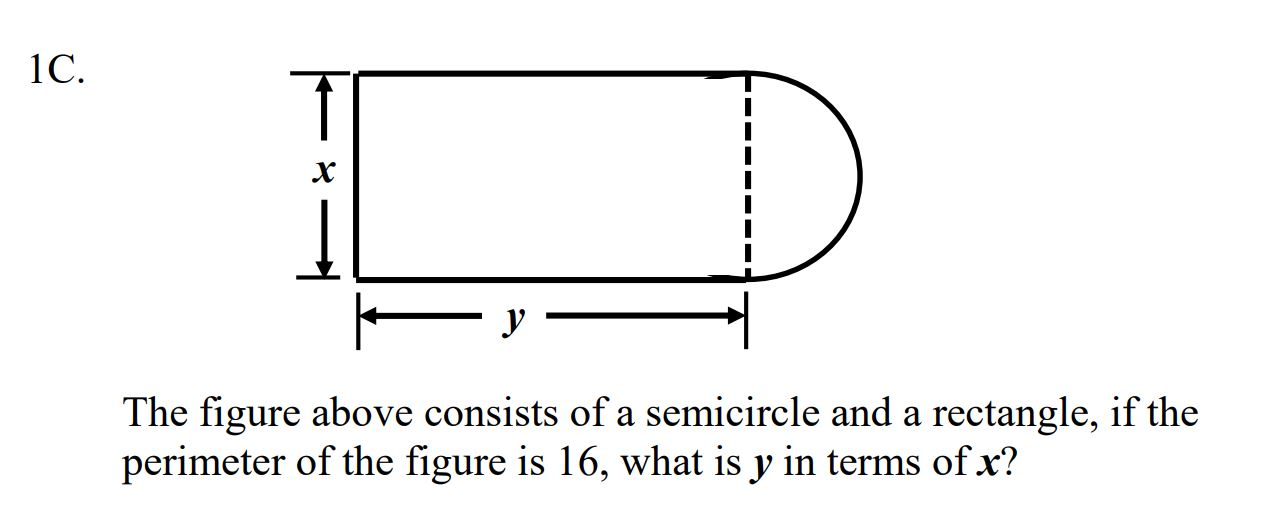# 1C. х У The figure above consists of a semicircle and a rectangle, if the perimeter of the figure is 16, what is y in terms ofx?

Question

How to solve for this equationhelp_outlineImage Transcriptionclose1C. х У The figure above consists of a semicircle and a rectangle, if the perimeter of the figure is 16, what is y in terms ofx? fullscreen

### Want to see this answer and more?

Experts are waiting 24/7 to provide step-by-step solutions in as fast as 30 minutes!*